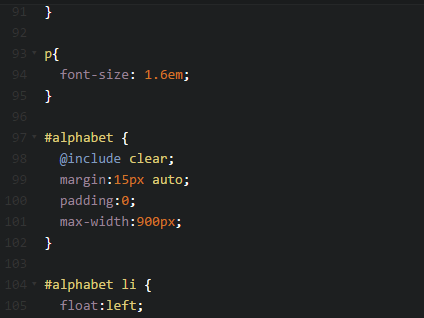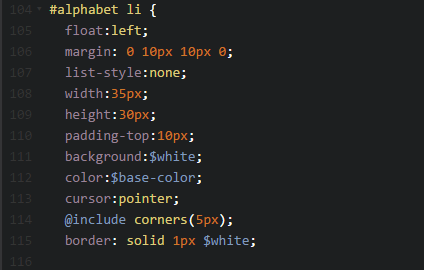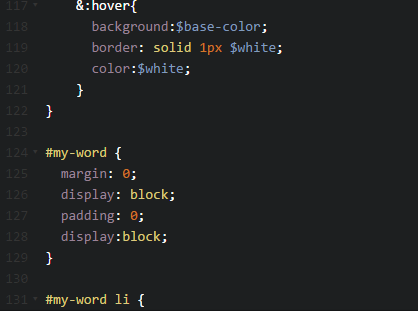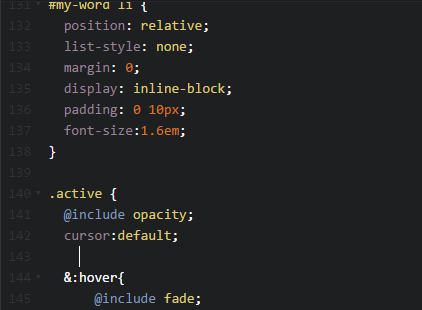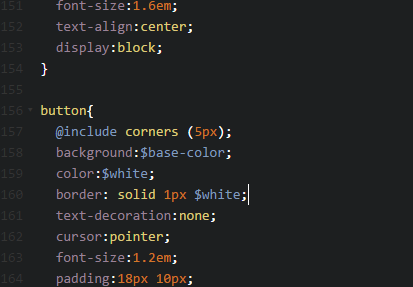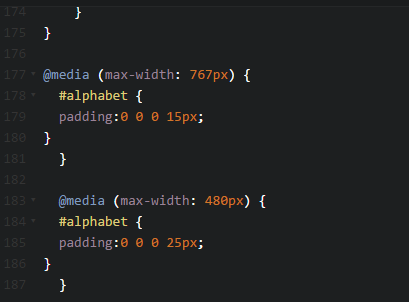# Answered! Consider a steady, frictionless one-dimensional flow of water through a nozzle. Use collocated grid as shown in the…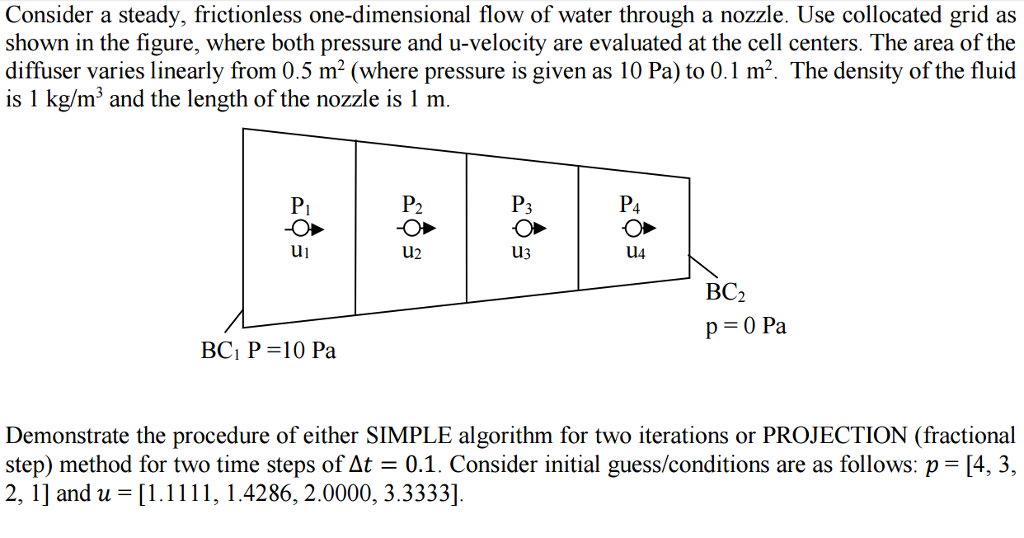Consider a steady, frictionless one-dimensional flow of water through a nozzle. Use collocated grid as shown in the figure, where both pressure and u-velocity are evaluated at the cell centers. The area of the diffuser varies linearly from 0.5 m^2 (where pressure is given as 10 Pa) to 0.1 m^2. The density of the fluid is 1 kg/m^3 and the length of the nozzle is 1 m. Demonstrate the procedure of either SIMPLE algorithm for two iterations or PROJECTION (fractional step) method for two time steps of Delta t = 0.1. Consider initial guess/conditions are as follows: p = [4, 3, 2, 1] and w = [1.1111, 1.4286, 2.0000, 3.3333].